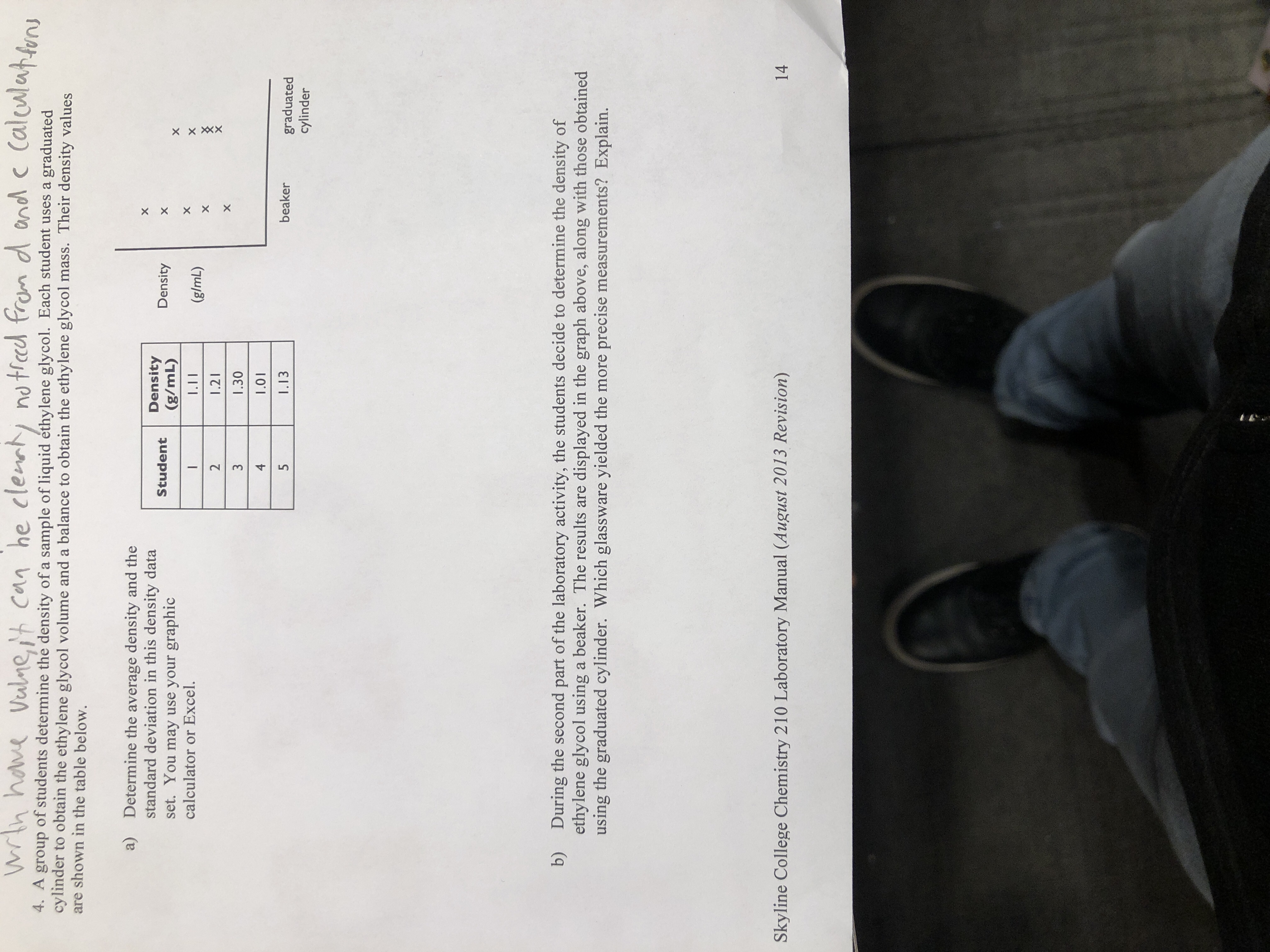# he clentyMh haveno ted from d ond c CalculatnVulneit can4. A group of students determine the density of a sample of liquid éthylene glycol. Each student uses a graduatedcylinder to obtain the ethylene glycol volume and a balance to obtain the ethylene glycol mass. Their density valuesare shown in the table below.a)Determine the average density and thestandard deviation in this density dataset. You may use your graphiccalculator or Excel.Density(g/mL)DensityStudentI.II(g/mL)21.211.3041.015I.13beakergraduatedcylinderb)During the second part of the laboratory activity, the students decide to determine the density ofethylene glycol using a beaker. The results areusing the graduated cylinder. Which glassware yielded the more precise measurements? Explain.displayed in the graph above, along with those obtainedSkyline College Chemistry 210 Laboratory Manual (August 2013 Revision)14XXXXX x x X X

Question
15 views

Question 4 A and Bhelp_outlineImage Transcriptionclosehe clenty Mh have no ted from d ond c Calculatn Vulneit can 4. A group of students determine the density of a sample of liquid éthylene glycol. Each student uses a graduated cylinder to obtain the ethylene glycol volume and a balance to obtain the ethylene glycol mass. Their density values are shown in the table below. a) Determine the average density and the standard deviation in this density data set. You may use your graphic calculator or Excel. Density (g/mL) Density Student I.II (g/mL) 2 1.21 1.30 4 1.01 5 I.13 beaker graduated cylinder b) During the second part of the laboratory activity, the students decide to determine the density of ethylene glycol using a beaker. The results are using the graduated cylinder. Which glassware yielded the more precise measurements? Explain. displayed in the graph above, along with those obtained Skyline College Chemistry 210 Laboratory Manual (August 2013 Revision) 14 XXXX X x x X X fullscreen
check_circle

Step 1

The average density can be calculated using the following data by adding the values of the given densities calculated by each of the students and then dividing the sum total by the total number of students.

Therefore, by making use of the excel and using the formula as shown below, one can make a table and find the average as shown below in the table.

Step 2

The average density of the given data is found to be 1.15 g/mL.

Step 3

The standard deviation in a given data is measure which is used to quantify the amount of variation that occurs in a given set of data values. ...

### Want to see the full answer?

See Solution

#### Want to see this answer and more?

Solutions are written by subject experts who are available 24/7. Questions are typically answered within 1 hour.*

See Solution
*Response times may vary by subject and question.
Tagged in

### Chemistry